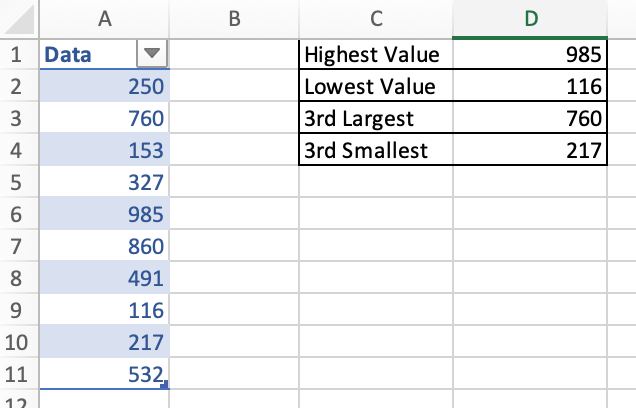### DEV4 Blog

• Home
• /
• Blog
• /
• Microsoft Excel: MIN, MAX, LARGE and SMALL Functions

### Microsoft Excel: MIN, MAX, LARGE and SMALL Functions

MAX, MIN, LARGE and SMALL are four functions of Excel which help with finding the range of data values in a dataset.

MAX allows you to find the highest value in a range of data using the formula =MAX(cell range to search). In the example below we used =MAX(A2:A11) to find the highest value in the range.

MIN allows you to find the lowest value in a data range using the formula =MIN(cell range to search). In the below example we used =MIN(A2:A11) to find the lowest value in the range.

LARGE does the same as the MAX function, however it also allows you to search for more than just the highest value, e.g., the 2nd highest, 14th highest etc. The formula for this is =LARGE(cell range to search, value desired), e.g., =LARGE(A2:A11, 3) to find the 3rd largest value in the range, as shown below.

SMALL is like LARGE, in that it does the same as the MIN function, however it allows for more than just the lowest value to be identified, e.g., 4th lowest. The formula used for this is =SMALL(cell range to search, value desired), e.g., =SMALL(A2:A11, 3) to find the 3rd smallest value in the range, as shown below.Published on 17 Mar 2021 by Joanna Hepburn